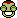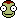## Recommended Posts

Hey guys,

the map view and the trajectories are very convenient but i would like to know a little bit more about the maths behind those trajectories. What are the mathematic formulas and functions to draw those trajectories and how much deltaV modify them.

Thanks for the help.

##### Share on other sites

These can get somewhat involved, and admittedly wikipedia doesn't always have things in the best format. The (ahem) textbook answer is Fundamentals of Astrodynamics. If you're looking purely for an online resource, @Ohiobob has done good work.

ok great thanks

##### Share on other sites
On 1/6/2019 at 8:19 PM, UmbralRaptor said:

The (ahem) textbook answer is Fundamentals of Astrodynamics.

Hey, look what I'm getting on my BirthdayThis looks interesting, thanks for sharing, even though I'm not the one who asked this##### Share on other sites
3 hours ago, Spaceception said:

Hey, look what I'm getting on my BirthdayThis looks interesting, thanks for sharing, even though I'm not the one who asked thisIt covers a reasonable amount of topics. Perhaps more importantly it's the only textbook I've seen where the US edition is reasonably priced. >_>

##### Share on other sites

Ok I'm starting to digg in, and I have a question about the form of gravity equation that is used.

Why do they keep a distance vector in the multiplyer and don't reduce the equation like this?:

##### Share on other sites
6 hours ago, Brainkite said:

Ok I'm starting to digg in, and I have a question about the form of gravity equation that is used.

Why do they keep a distance vector in the multiplyer and don't reduce the equation like this?:

think that since the rni isn't bold, it's a scalar, rather than a vector, which rni is. Vector division isn't really defined, so I think that this is dividing by the norm (or magnitude) of the vector, then multiplying by rni/||rni||, where the double pipes mean norm, which produces the "normalized" vector with a magnitude of 1, thus only preserving direction. Then the ||rni|| is moved over to the fraction on the left.

It seems like they also do this in 1.1.3 on pg 4, where they define Newton's law of universal gravitation as

Fg = - ((GMm)/(r^2)) * (r/r)

I'm not really sure why they would use unbolded variables as the magnitude though.

##### Share on other sites
1 hour ago, Mad Rocket Scientist said:

think that since the rni isn't bold, it's a scalar, rather than a vector, which rni is. Vector division isn't really defined, so I think that this is dividing by the norm (or magnitude) of the vector, then multiplying by rni/||rni||, where the double pipes mean norm, which produces the "normalized" vector with a magnitude of 1, thus only preserving direction. Then the ||rni|| is moved over to the fraction on the left.

It seems like they also do this in 1.1.3 on pg 4, where they define Newton's law of universal gravitation as

Fg = - ((GMm)/(r^2)) * (r/r)

I'm not really sure why they would use unbolded variables as the magnitude though.

This is a moderately common convention in various physics texts. I think it ends up being easier to typeset than getting the arrows and hats over the vectors right?

Edited by UmbralRaptor

## Join the conversation

You can post now and register later. If you have an account, sign in now to post with your account.
Note: Your post will require moderator approval before it will be visible.